Get instant live expert help with Excel or Google Sheets“My Excelchat expert helped me in less than 20 minutes, saving me what would have been 5 hours of work!”

#### Post your problem and you’ll get expert help in seconds.

Your message must be at least 40 characters
Our professional experts are available now. Your privacy is guaranteed.

# How to Use Norm.dist and Norm.s.dist functions

We can execute a norm.dist. in excel by using the mean and standard deviation of a given data set. This tutorial will teach us how to calculate normal distribution in excel.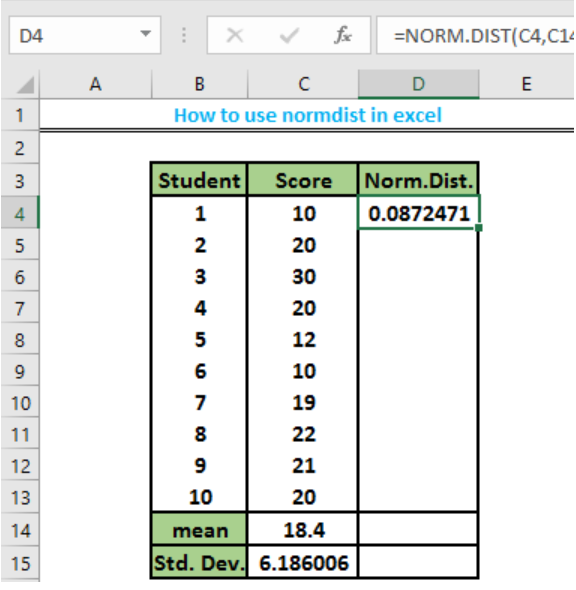Figure 1: Normal Distribution

## How to Use the Excel norm.dist

• We will create a table of data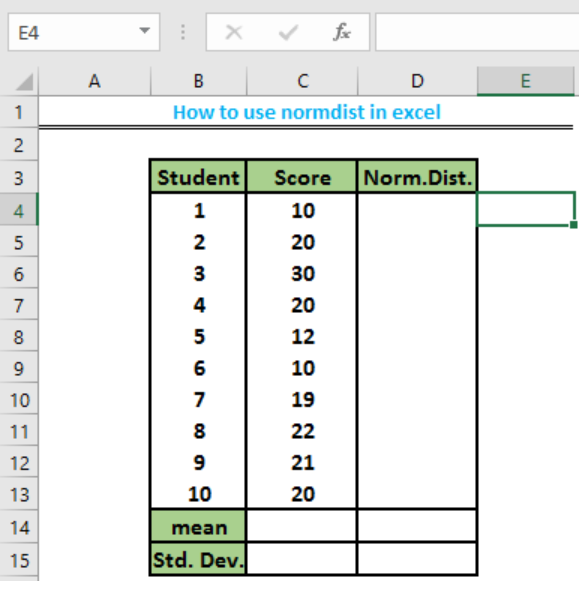Figure 2: Table of data

• We will click on Cell C14 to calculate the mean of our data. We will type this formula =AVERAGE(C4:C13) into the cell to automatically calculate the average.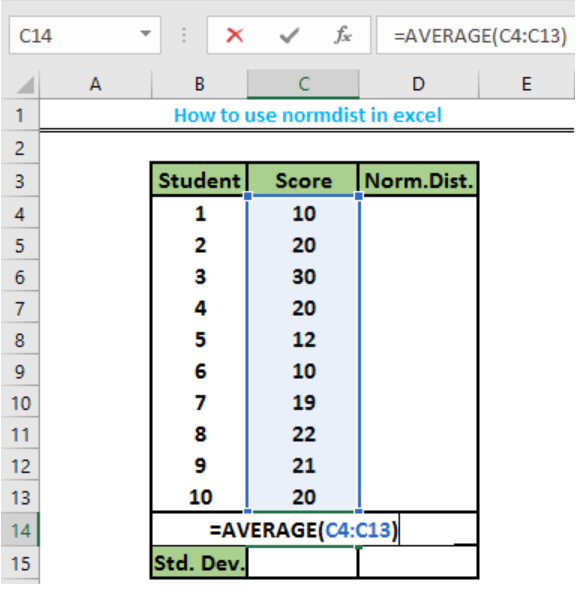Figure 3: Formula for Average

• This will give us the average value of our data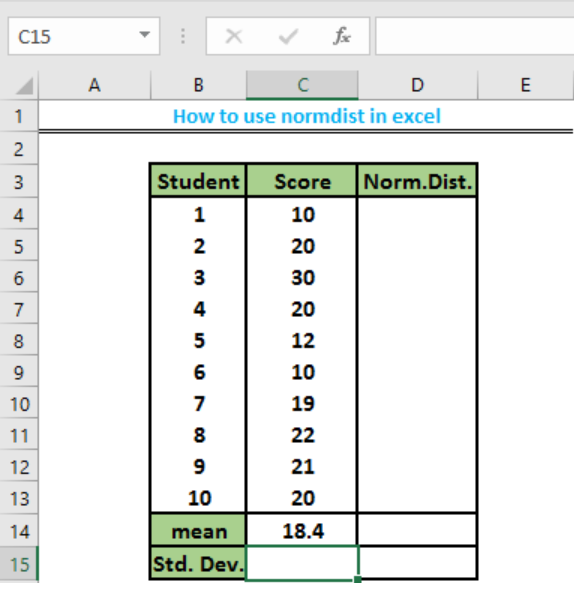Figure 4: Average or Mean value of our data

• We will now calculate the standard deviation of our data set by typing this formula `=STDEV(C4:C13)` into Cell C15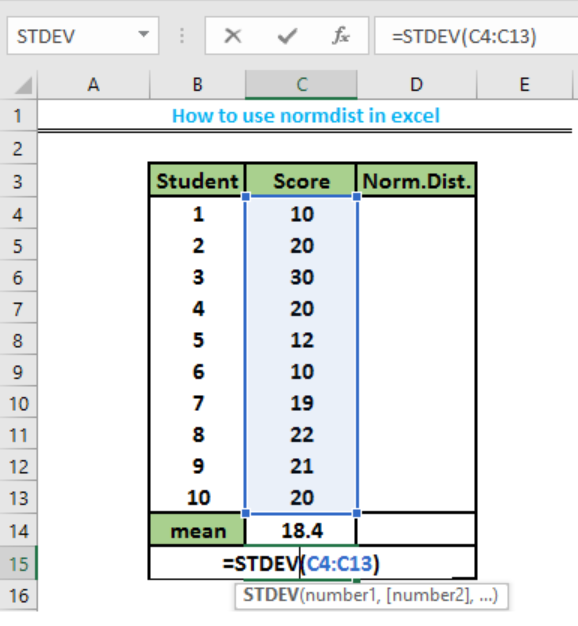Figure 5: Standard deviation formula

• This will give us the answer to our standard deviation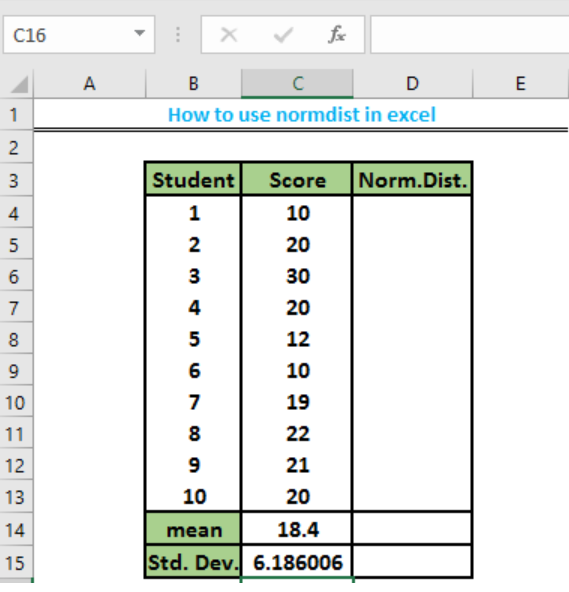Figure 6: Standard deviation

• We will now determine the normal distribution of our data set by using clicking on Cell D4. We can use this formula `=NORM.DIST(C4,C14,C15,TRUE)` or `=NORM.DIST(C4,C14,C15,FALSE)`. We can also follow this procedure by selecting the fx function above the worksheet, this will open a dialogue box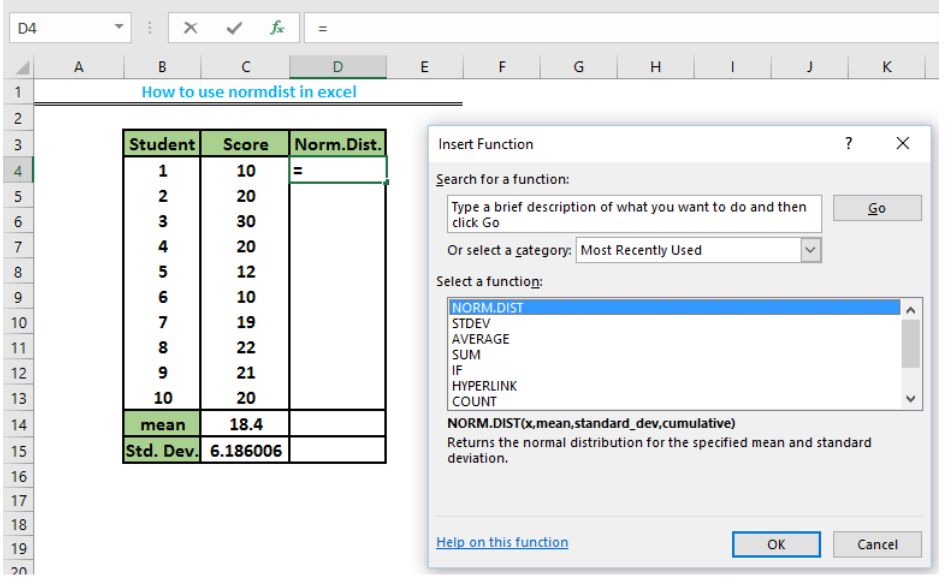Figure 7: Dialogue box for fx

• We will type normal distribution in the search option, we will now select norm.dist. This will bring out a dialog box, requesting for X, Mean, Standard deviation and cumulative.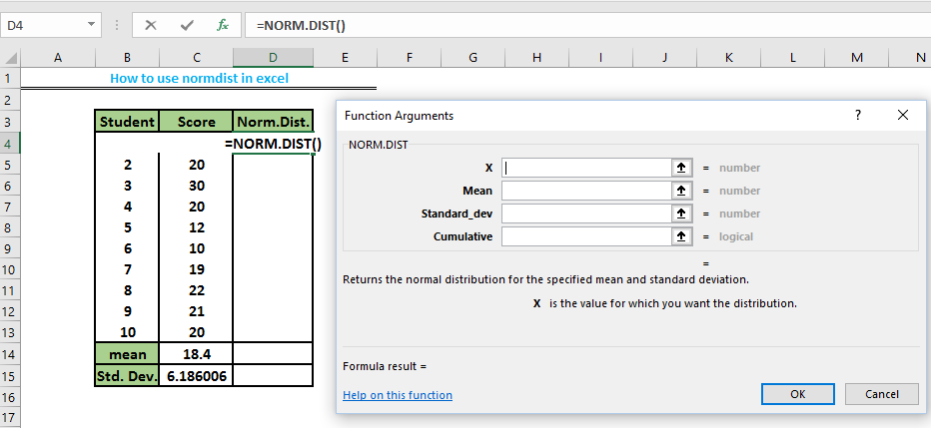Figure 8: Normal distribution dialog box

• We will now input the information; where X is score of the student in cell C4, we will click on cell C4, then we will click on cell C14 to input the mean, then we will click on cell C15 to input the Standard deviation. We will now type TRUE if we want the cumulative distribution function or FALSE in the cumulative box if we want the probability density function.

TRUE means the probability that the student scored exactly the score in Column C or less than the score in Column C

FALSE means the probability that the student scored exactly the score in Column C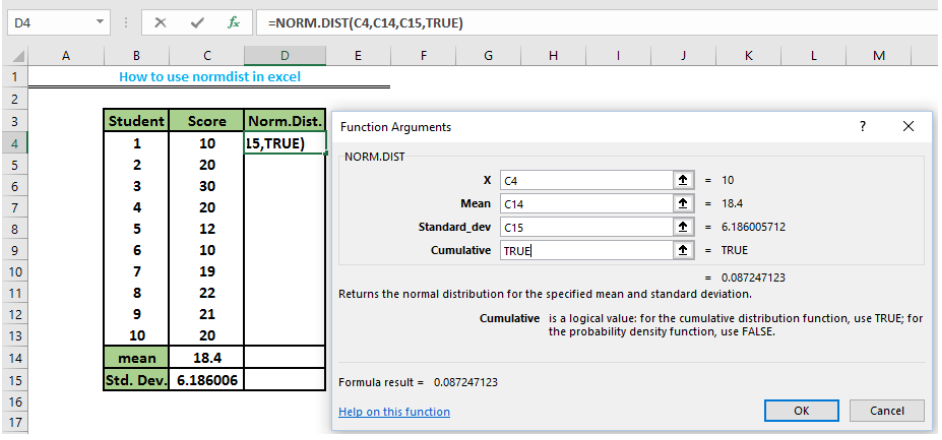Figure 9: Normal Distribution

• This will return the result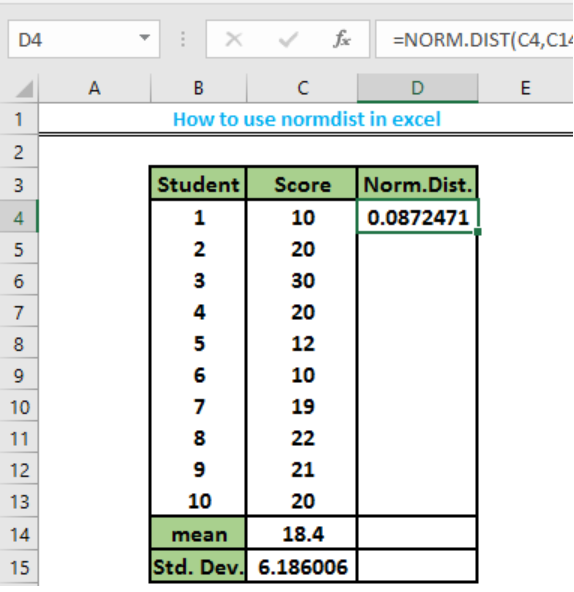Figure 10: Normal Distribution

• We can repeat the same procedure for the remaining data set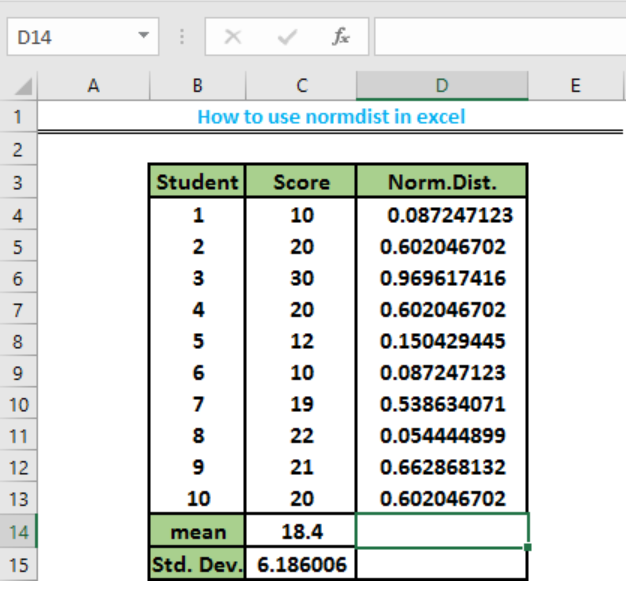Figure 11: Normal distribution

## How to Use the Norm.s.dist Function

Norm.S.Dist is different from the Norm.Dist function. Norm.S.Dist is used to convert Z values to expected probabilities of a particular occurrence in a data set. Z is the standard deviation distance from the original mean value.

• To perform Norm.S.Dist, we will create an additional column for the standardized value and the Norm.S.dist resultsFigure 12: Columns for Standardized Value and Norm.S.Dist

• To calculate the standardized value (Z) for Cell E4, we will click on the fx operation and type into the search box STANDARDIZE, then we will select the standardize option, which will open a dialog box. This will request for the X, mean and standard deviation values.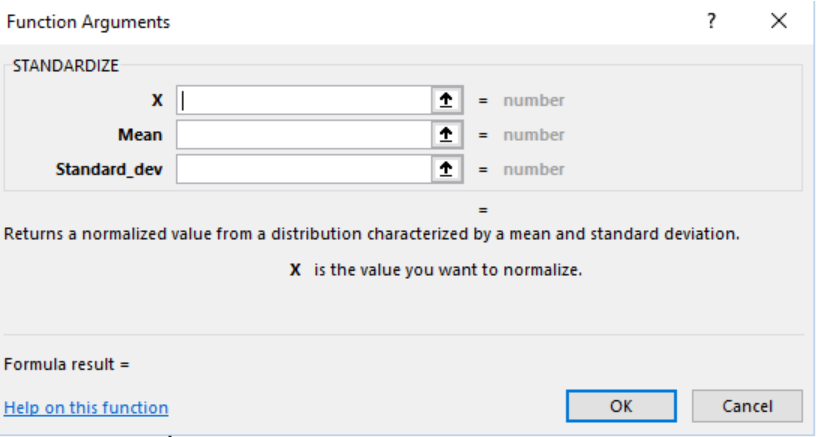Figure 13: Dialog box for Standardize value

• We will now input the data requested to calculate the standardized value for Cell E4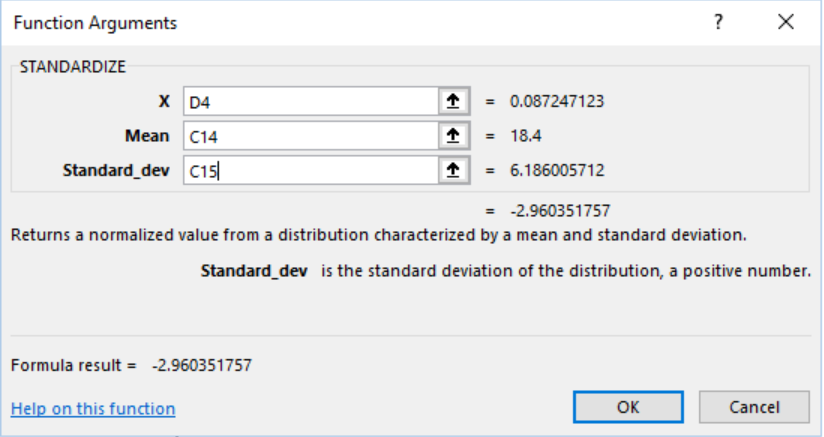Figure 14: Values for calculating Z

• This will return the result in Cell E4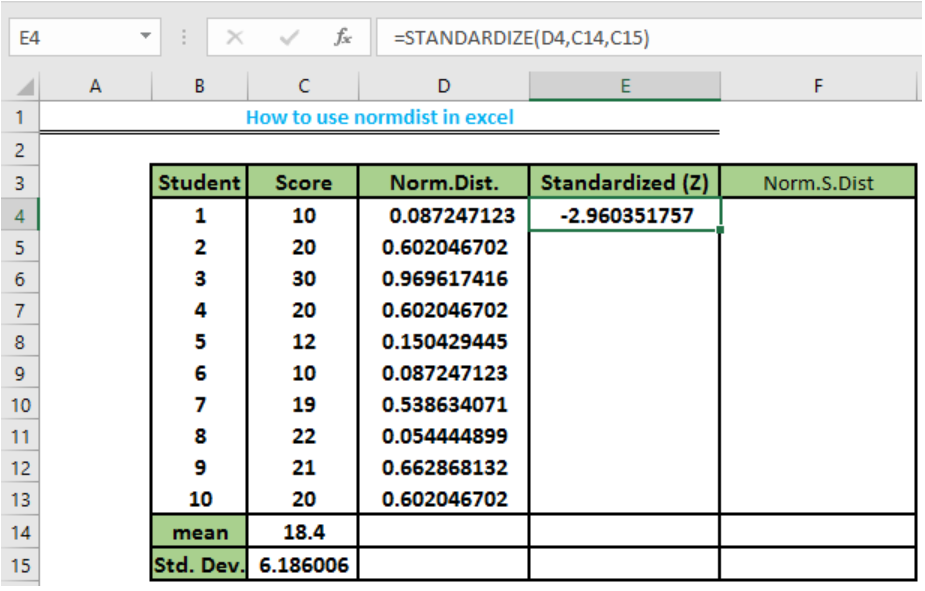Figure 15: Standardized value

• We will input the value of Z, then we will type TRUE if we want the cumulative distribution function or FALSE in the cumulative box if we want the probability density function.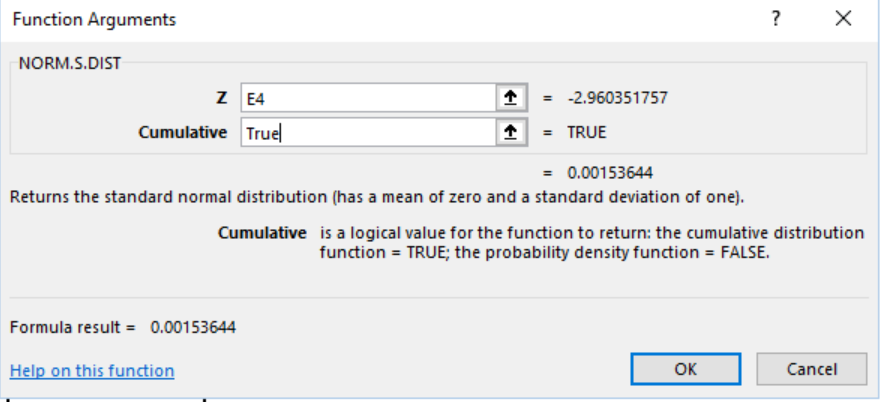Figure 16: Inputting the data for Norm.S.Dist

• We will now click OK to display the result in Cell F4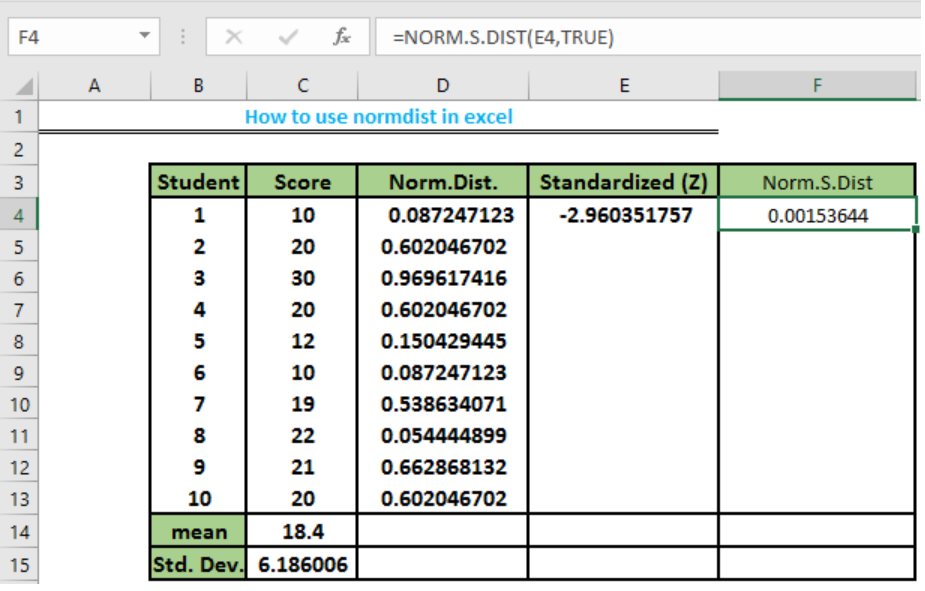Figure 17: Result for Norm.S.Dist

• We can repeat this operation for the remaining data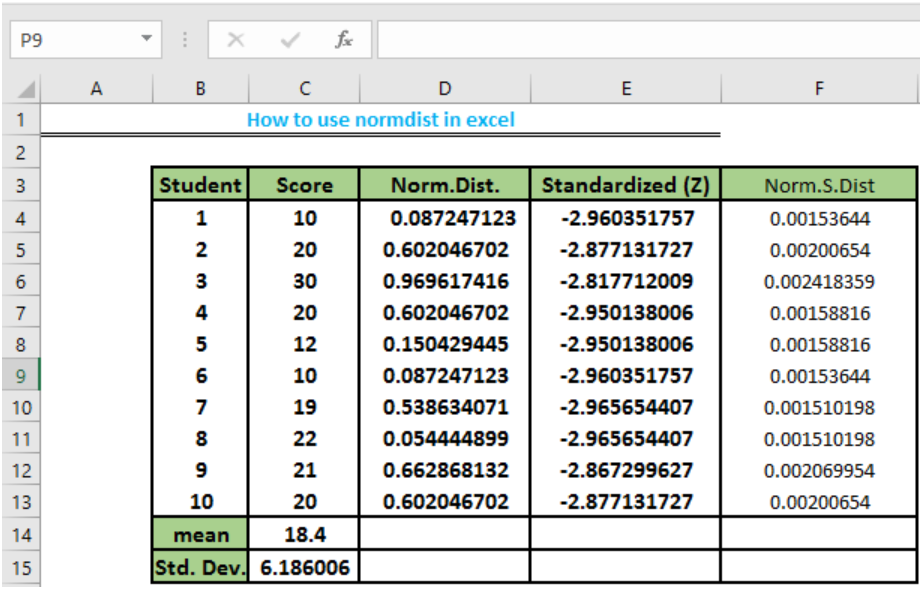Figure 18: Norm.S.dist

## Instant Connection to an Expert through our Excelchat Service

Most of the time, the problem you will need to solve will be more complex than a simple application of a formula or function. If you want to save hours of research and frustration, try our live Excelchat service! Our Excel Experts are available 24/7 to answer any Excel question you may have. We guarantee a connection within 30 seconds and a customized solution within 20 minutes.